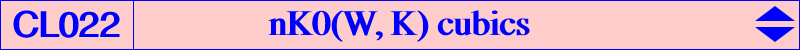Given an isoconjugation with pole W(p : q : r), the locus of point P such that the pedal triangles of P and its W-isoconjugate P* are parallelogic is nK0(W, K = X6), if we omit the line at infinity and the trilinear polar of the isotomic conjugate of the isogonal conjugate of W. See another generalization at K024 property 11. All these cubics contain the centers of the 3 Apollonian circles. K148 is the only cubic of this type which is circular and Kjp = K024 is the only cubic of this type which is a K60 and also which is isogonal. K149 is the isotomic cubic of the class. K147 is an example of singular cubic of the class. See below. The cubic is a nK0+ if and only if W lies on K150. See K151 for example. Compare this type of cubic with CL021. The barycentric equation of nK0(W, X6) is : ∑ a^2 x (r y^2 + q z^2) = 0. Each nK0(W, K) can also be seen as the locus of point P such that P and P* are conjugated with regard to the circumcircle. More generally, each nK0(W, R) is the locus of point P such that P and P* are conjugated with regard to the circum-conic with perspector R. The following table gives a selection of nK0(W, K) already mentioned in CTC.W cubic or X(i) on the cubic remarks X(6) K024 Kjp cubic isogonal nK60+ X(110)^2 K147 nodal X(50) K148 circular X(2) K149 isotomic X(99) K151 X(187) K604 nK0+ X(14273) K608 central X(385) K740nK0(W, K) is a singular cubic if and only if either • W lies on the trilinear polar of X(32) in which case the cubic splits into the Lemoine axis and the circum-conic which is its isoconjugate, • W is the barycentric square of a point N on (O) which is the node. See K147 for instance, also nK0(X1252, X6), nK0(4590, X6) with nodes X(100), X(99) respectively.